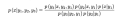# How to apply the the conditional probability and chain rule formulas in a multivariable case?

#### Fra.Z.

Joined Jun 27, 2022
1
I'm learning about Bayesian Fusion and have a question regarding an expression that I wasn't able to prove.

It poses the following statement:

Assume 1,2,3y1,y2,y3 are three observations which are neither independent nor conditionally independent given x with each other. Prove that the following expression holds:I'm trying to prove the expression above using the formulas related to conditional probability, chain rule, but couldn't really conclude anything. Unfortunately, given that the variables are not independent nor conditionally independent, formulas such as the Bayes formula, etc.. can't be used, since they assume indipendence between variables.

(I tried to look for clues on the internet for 2 weeks already, but without meaningful results.)

If somebody could provide a breakdown of the calculations of the rule in this problem that would be great.